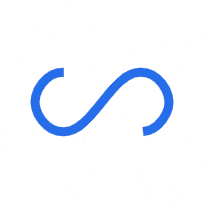# Categories of data structures

Data structures are generally classified into primitive and non-primitive data structures. Different categories of data structures are shown in the figure.

## Primitive data structures

Basic data structures that cannot be further divided is called primitive data structures.

## Non primitive data structures

Data structures that can be used for other complex storages are called non-primitive data structures.

Non-primitive data structures are again classified as linear and non-linear data types. A data structure is said to be linear if its elements form a sequence. Data structures like Array, Stack, Queue and linked list organizes data in linear order. A non-linear data structure is one in which its elements are not arranged in sequence. Trees and graphs are widely used non-linear data structures. It represents a hierarchical relationship between individual data elements.

### Arrays

A linear array or a one-dimensional array is the simplest type of data structure. It is a list of a finite number, n, of data elements of a similar type. These elements are represented by a set of consecutive numbers respectively. If A is the array name, then the array elements are represented as any of the following notations.A two-dimensional array is a collection of similar data elements where each element is referenced by two subscripts.A linked list is a collection of data elements whose order is not given by their physical location in memory. It consists of nodes that contain data and link to the next node. The structure allows easiness in insertion and deletion operations. The last nodes are linked to NULL and signify the end of the list.

### Trees

Some data contains a hierarchical relationship between various elements. These data are represented in the form of a Rooted tree graph or simply Tree. Here node A is called the root of the tree.

### Stack

Stack, LIFO(Last In First Out) system, is a linear data structure in which insertion(PUSH) and deletion(POP) are restricted to one endpoint called TOP.

### Queue

A queue is a FIFO(First In First Out) system, in which insertion(ENQUEUE) can take place only at an end called REAR and deletion(DEQUEUE) can take place only at another end called FRONT.

### Graphs

Sometimes data contain relationships that are not hierarchical in nature. This type of relationship can be expressed in the form of a graph data structure.

Subscribe
Notify of